Email us to get an instant 20% discount on highly effective K-12 Math & English kwizNET Programs!

#### Online Quiz (WorksheetABCD)

Questions Per Quiz = 2 4 6 8 10

### Geometry3.8 Rhombus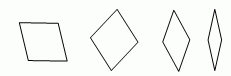Properties of Rhombus: The diagonals in a rhombus bisect each other. Each diagonal of a rhombus divides it into two congruent triangles. Opposite angles of a rhombus are equal and the sum of any two adjacent angles is 180�. In a rhombus the diagonals bisect each other at right angles, that is, the diagonals are perpendicular to each other and bisect each other. THEOREMS A quadrilateral is a rhombus, if and only if it has four congruent sides.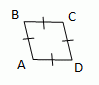AB @ BC @ CD @ DA A parallelogram is a rhombus, if and only if its diagonals are perpendicular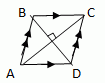AC ^ BD A parallelogram is a rhombus, if and only if each diagonal bisects a pair of opposite angles.Directions: Read the above review points carefully and answer the following questions: Explain the different theorems of a rhombus, in your own words, with examples. Illustrate each of the above review points by drawing different rhombus. If one of the angles of a rhombus is 2 times the adjacent angle, then what will be the value of the angle opposite to it? Give reasons. A side of a rhombus is given by 16 cm. What will be the value of half of the other side? Give reasons. Question: The diagonals of rhombus ABCD intersect at O. Given that measure of �BAC is 530 and DO = 8, Find the m�ABO, m�ABC, m�BCO, m�BCD, m�CDO, m�CDA, m�AOB, m�BOC, m�AOD, AO, AC, BD (Hint: Use pythagorean theorem to find the measures of angles)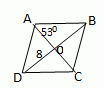Q 1: The length of each side of the rhombus shown is ____.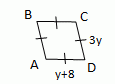4123 Q 2: The value of �E in the given rhombus is __________.60�90�45� Q 3: The opposite sides of a rhombus are given by 6 cm, 6 cm and 8 cm. Then the third side will be __________.12 cm8 cm6 cm Q 4: Each diagonals of a rhombus divides it into two __________.congruent trianglessimilar trianglesright angled triangles Q 5: The two adjacent angles of a rhombus have degree measures 60 and 30.FalseTrue Q 6: One of the diagonals of a rhombus is given by 10 cm. Then it divides the rhombus into two __________.perpendicular bisectorscongruent triangleshalves is 5 cm each Q 7: The value of side DC if side AB is given by 12.5 cm, is __________.7.5 cm15 cm11 cm Q 8: The diagonals of a rhombus bisect at __________.right anglesobtuse anglesacute angles Question 9: This question is available to subscribers only! Question 10: This question is available to subscribers only!